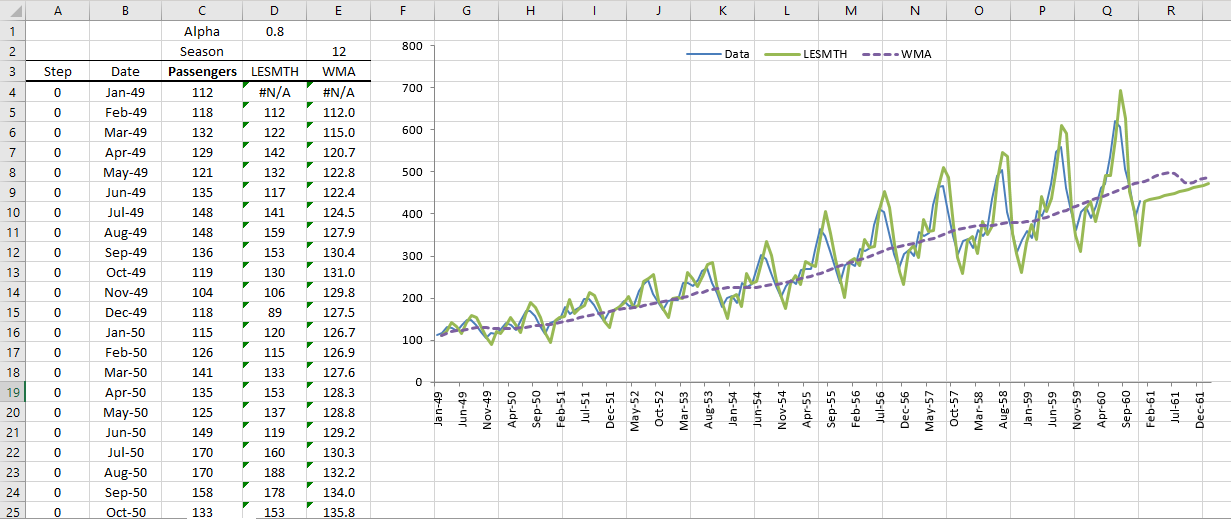# Brown's Linear Exponential Smoothing in Excel (LESMTH)

Check out Brown's Linear Exponential Smoothing (LESMTH) tutorial in NumXL below. The playlist contains tutorials that teach you how to utilize the optimization switch and calculate with seasonal decomposition.

Optimization On Optimization Off Decomposition

## Optimization On

In this video, we show you how to use Brown's Linear Exponential Smoothing in NumXL with an optimization switch for smoothing factors.

Video script

Scene 1:

Hello and welcome to the exponential smoothing tutorial series. In this tutorial we will resume the demonstration of Brown's linear exponential smoothing function, and use the built-in optimizer in NumXL. For the sample data we are using the same weekly demand volume data of a hypothetical product.

Let's begin by selecting cell D9. examine the cell formula in the formula toolbar. Notice we already have a call for the linear exponential smoothing function, so press F2 to edit. Click on the FX button found on the left side of the equation toolbar, this will invoke the function arguments dialog box for the linear exponential smoothing function.

Scene 2:

Notice that the cell D2, is used for the optimize function, so we don't need to change the formula just the value in D2 to turn on the optimizer. Please click cancel to go back to your worksheet.

Scene 3:

Select the D2 cell and type in true or 1 then hit enter when done.

Scene 4:

Since we have the automatic calculation on all of the values of the smooth time series are recalculated using optimal smoothing factor known as alpha. Notice the change in mean squared error mean, absolute scaled error and symmetric mean absolute percentage error.

The calibrated linear exponential smoothing has a 21 percent lower mean absolute error than that of a naive reference model. Note that the function calculates the optimal value for alpha using the available information or data as a training set. The available data increases with time so the function calculates a new value each time.

What about the alpha value in D1? Well when the optimizer flag is turned on the function uses the value of alpha as a starting value for the optimizer.

Now let's examine the value of the smoothing parameter at each period. Select the E10 cell and start typing in the function =LESMTH(. Click on the FX button found on the left side of the equation toolbar, this will invoke the function arguments dialog box for the linear exponential smoothing function.

Scene 5:

Then specify the input cell range, this is the same cell range we used earlier for the forecast in column D. Lock the starting cell in the input range by pressing F4, enter a value of true or one in the order field.

Scene 6:

For the initial value of alpha let's use the value in D1, then lock the cell by pressing F4. For the optimized switch let's use the value in cell D2 up the cell by pressing F4.

Scene 7:

Set the forecast time to zero or set the value in cell A10, and lock the cell for column movement. For the return type in one for returning the value of the smoothing parameter.

Scene 8:

The built-in optimizer requires a few non-missing observations to run or it will return the starting alpha value as it is the case here.

Scene 9:

Now copy the formula to the cells below it.

Scene 10:

The value of the smoothing parameter at each period is calculated, let's plot the values of the smoothing parameter versus time. The graph shows the volatility of the computed alpha values throughout the sample in the first year. In a second year the value of the smoothing factor declines to very low levels then picks up to three point five percent.

So what's going on? Well the SSE function seems to have multiple minima and their values are comparable close. Earlier in the sample data the first minimum seem to have a lower value and as we have more observations the second minima have lower value and so it was picked up by the optimizer. To see for yourself simply compute the SSE when alpha is 3.5% and for 0.6%.

Does it matter which alpha I use? We prefer a stable alpha over time so we can designate part of the sample data as a training set and use the rest to assess the forecasting power. In this scenario we'd use the first 85 observations, about 1.5 years as a training set and leave nine observations for measuring forecast accuracy.

In a follow-up tutorial we will decompose the input data into two components, level and trend, and query the linear exponential smoothing for their values.Scene 11:

That's all for now, thank you for watching!